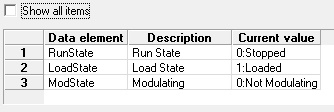# Using references to display a packed word value

August 3, 2015 / 0 comments / in General  / by Ryan Ackerman

Have you ever run into a situation where a single value on a device could represent multiple values at the same time? How would you display a nice string representation for all the potential values? I recently saw this problem solved on an Emerson device where a TLP contained multiple values packed into a single byte. The solution used a combination of multiple reference DEIDs and a few associated enumerations.

First define the DEID that represents the data you want to unpack. In this example the data is arbitrary and could be any data from any EIE type; I have used an Emerson device. Notice that I have marked this DEID as hidden. The hidden attribute allows me to hide the raw value, which could be difficult to understand.

`<WordValue desc="Packed Word Value" type="ui2" tlpType="98" tlpLog="2" tlpParm="30" hidden="true"/>`

Next define the references to the packed value. These references will extract the specific content based on the bit values in the source word value and then display a nice string representation of the result value.

The bMsk attribute allows me to do a bitwise AND operation on the value and then a bit shift to move the result value all the way to the right. Alternatively I would have used the bPos attribute depending on how I am analyzing the source value. The result of this specific operation will be either a 1 or a 0 if the second bit is set or unset. Based on that result value I can apply an enumeration to the value to display a nice string. Notice that I have also included a default value in the event I get a value I am not expecting.

`<RunState desc="Run State" type="string" ref="WordValue" bMsk="0x0012" mapVal="RSMap">`

`    <RSMap>`

`        <e0 value="0:Stopped"/>`

`        <e1 value="1:Running"/>`

`        <defVal value="Unknown Run State"/>`

`    </RSMap>`

`</RunState>`

Now I can repeat the process for each part of the packed word value I want to analyze.

`<dgElements>`

`    <WordValue desc="Packed Word Value" type="ui2" tlpType="98" tlpLog="2" tlpParm="30" hidden="true"/>`

`    <RunState desc="Run State" type="string" ref="WordValue" bMsk="0x0002" mapVal="RSMap">`

`        <RSMap>`

`            <e0 value="0:Stopped"/>`

`            <e1 value="1:Running"/>`

`            <defVal value="Unknown Run State"/>`

`        </RSMap>`

`    </RunState>`

`    <LoadState desc="Load State" type="string" ref="WordValue" bMsk="0x0004" mapVal="LUMap">`

`        <LUMap>`

`            <e0 value="0:Unloaded"/>`

`            <e1 value="1:Loaded"/>`

`        </LUMap>`

`    </LoadState>`

`    <ModState desc="Modulating" type="string" ref="WordValue" bMsk="0x0010" mapVal="MODMap">`

`        <MODMap>`

`            <e0 value="0:Not Modulating"/>`

`            <e1 value="1:Modulating"/>`

`        </MODMap>`

`    </ModState>`

`</dgElements>    `

The result view in the editor will look something like this:## Related PostsPrevious PostPlaying Battleship with the HSSBlog post currently doesn't have any comments.
 {{com.name}}           {{com.blogCommentDateFormatted}}

• {{ error }}